### Home > PC > Chapter 9 > Lesson 9.2.2 > Problem9-87

9-87.
1.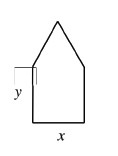A farmer with an eye for the unusual has decided to build a pentagonal enclosure whose shape will be a rectangle with an equilateral triangle sitting on top of it. (See figure at right.) The perimeter is 100 meters. Homework Help ✎

1. Label all sides.

2. Find y in terms of x.

3. Find the area of the figure as a function of x.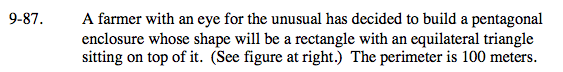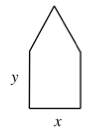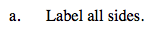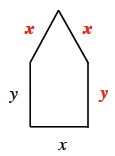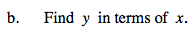2y + 3x = 100
2y = 100 − 3x

$y=\frac{100-3x}{2}$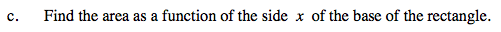Areatotal = Arearectangle + Areatriangle

To find the area of the triangle, drop an altitude forming two congruent triangles. The diagonal will be 'x'.

$\footnotesize \text{Use the Pythagorean Theorem to find the height. The area will be }\frac{(\text{height})(\text{base})}{2}.$